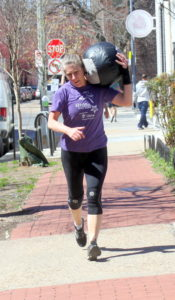## Tuesday, May 7, 2019

### Tuesday, May 7, 2019With a continuously running clock, you have 15 minutes to complete 75x russian swing @ 70/55-lbs. and then in the remaining time as many rounds as possible of a 400m run and 75x double under.

### 1 Comment

1.–Scores–
Al C: 2 + 400m
Charles B.: 4 rounds, 60 du (sub: 1 min double under attempts)
Rami B.: 3 rounds + 200m (45-lbs.; sub: 1 min double under attempts)
Adrian A.: 2 rounds + 200m (35-lbs.)
Tamra F.: 3 rounds, 10 du (25-lbs. sub: 100m run, 1 min double under attempts, cap 10 min)
Borja G.: 3 rounds + 400m
Alex M.: 3 rounds + 300m (55-lbs)
Hari P.: 3 rounds + 100m
Aaron B.: 3 rounds + 300m (sub: 20 lunges)
Kevin K.: 3 rounds (45-lbs.)
Andrew Z.: 3 rounds + 150m
Jon K.: 2 rounds + 400m, 26 du (35-lbs. sub: 1 min double under attempts)
Israel S.: 2 rounds + 46 du (53-lbs.)
Anna G.: 3 rounds + 100m, 45 du (25-lbs.)
Jason O.: 3 rounds + 75m
Sean F.: 2 rounds + 26 du, 143 du (sub: 1 min double under attempts)
Holmes H.: 2 rounds + 30″ du practice, 25 du (25-lbs.)
Will H.: 3 rounds, 6 du (55-lbs.; sub: 1 min double under attempts)
Walt C.: 2 rounds + 32 du (55-lbs.)
David C.: 2 rounds + 2 cal (55-lbs.; sub: 15 cal bike)
Ben P.: 4 rounds (35-lbs.; sub: 25 quick steps)
Edward: 3 rounds
Adrian P.: 3 rounds, 75 du (55-lbs.)
Zach D.: 3 rounds + 150m, 142 du (45-lbs.)
Jerry I.: 3 rounds, 37 du (45-lbs.)
Anu B.: 2 rounds + 29 (55-lbs.)
Colin S.: 3 rounds + 200m, 12 du (45-lbs.)
Dave R.: 5 rounds +7 cal (sub: 15 cal bike, 5x burpee, 10x step-up, 24″)
Ralph B.: 3 rounds + 200m 84 Du (45-lbs.)
Francisco L.: 3 rounds + 75m (55-lbs.)
Andrew B.: 2 rounds + 70 du (45-lbs.)
Drew P.: 3 rounds + 150m (55-lbs.)
Melody F.: 2 rounds + 61 du
Joel P.: 3 rounds (55-lbs.)
Kate G.: 3 rounds, 69 du (35-lbs.)
Simon B.: 2 rounds + 250m, 6 du (25-lbs.)
Diana G.: 2 rounds (25-lbs.)
Lisa C.: 3 rounds, 99 du (45-lbs.)
Buffy M: 2 rounds + 300m (sub: 10 cal bike)
Raymond: 3 rounds + 75m
Bill M.: 3 rounds (45-lbs.)
Tommy W.: 2 rounds + 400m, 57 du (55-lbs.)
Jin D.: 3 rounds + 30x du (45-lbs.)
Chris H.: 3 rounds + 200m
Alli P.: 3 rounds + 250m
Joya TD.: 3 rounds, 132 du (45-lbs.)
Jason S.: 2 rounds + 400m, 33 du (55-lbs.)
Charlie S.: 3 rounds, 187 du (55-lbs.)
John La.: 2 rounds + 22 du (55-lbs.)
Anna Go.: 2 rounds + 125m, 18 du (45-lbs.)
Michael H.: 3 rounds (35-lb. db, 10-lb. db; sub: 50x body jump, 24″ + amrap; 24x hps + 16x sa ohs + 10x cal bike)
Joe T.: 3 rounds + 50m
Charlie S.: 3 rounds, 72 du
Josh M.: 2 rounds + 400m
Dan C.: 2 rounds + 400m, 82 du
John S.: 2 rounds + 300m, 95 du (45-lbs.)
Tara S.: 2 rounds + 26x du
Luke B.: 4 rounds, 63 du (45-lbs.)
Seth C.: 3 rounds, 46 du (55-lbs.)
Chris C: 3 rounds (sub: 400m row + 75x du + 25x sit-up throw w 14-lbs.)
Michail H.: 2 rounds + 200m, 22 du (55-lbs.)
Katie G.: 2 rounds + 350m, 20 du (45-lbs.)
Tarek K.: 2 rounds, 1 du (55-lbs.)
Andy G.: 2 rounds + 200m, 28 du (55-lbs.)
Hirofumi J.: 2 rounds, 89 du (35-lbs.)
Max M.: 2 rounds + 250m, 75 du
Lily S.: 2 rounds + 200m, 20 du (45-lbs.)
Aron S.: 2 rounds, 10 du (45-lbs.)
Mark Co.: 2 rounds + 175m (55-lbs.)
Patrick J.: 2 rounds + 320m (55-lbs.)
Bill G.: 2 rounds + 55x du (sub: 20-cal bike)
Terry A.: 2 rounds (20-lbs., 15-lb. plate; sub: superman, shoulder press)
Cristian D.: 3 rounds, 50 du (35-lbs.; sub: kb high pull)
Krystin H.: 1 rounds + 6x squat (sub: 100 lunge, 25x sir squat)
Seth B.: 2 rounds + 250m, 29 du (55-lbs.)